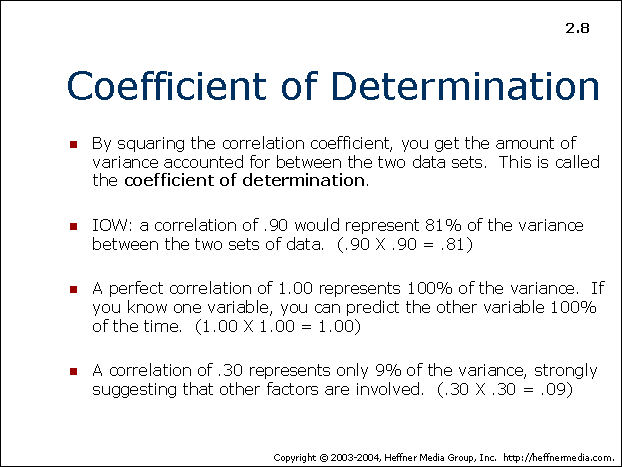advertisement

# 08## Coefficient of Determination

• By squaring the correlation coefficient, you get the amount of variance accounted for between the two data sets. This is called the coefficient of determination.
• IOW: a correlation of .90 would represent 81% of the variance between the two sets of data. (.90 X .90 = 0.81)
• A perfect correlation of 1.00 represents 100% of the variance. If you know one variable, you can predict the other variable 100% of the time. (1.00 X 1.00 = 1.00)
• A correlation of .30 represents only 9% of the variance, strongly suggesting that other factors are involved. (.30 X .30 = .09)